# GMAT Math : DSQ: Understanding arithmetic sets

## Example Questions

2 Next →

### Example Question #11 : Sets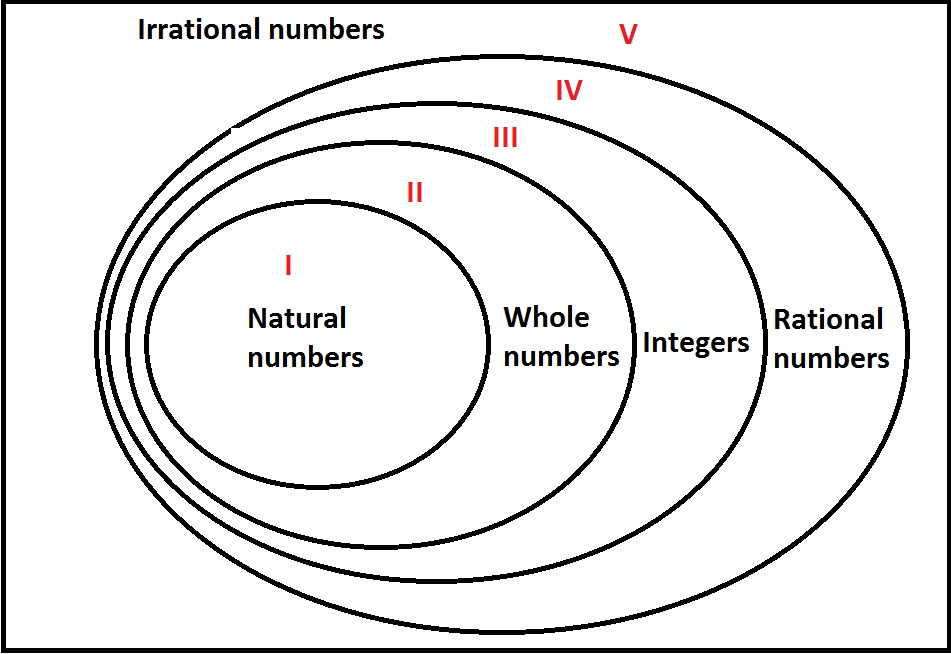Examine the above diagram, which shows a Venn diagram representing the sets of real numbers.

If real numberwere to be placed in its correct region in the diagram, which one would it be - I, II, III, IV, or V?

Statement 1: If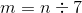, thenwould be placed in Region IV.

Statement 2: If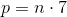, thenwould be placed in Region IV.

Statement 2 ALONE is sufficient to answer the question, but Statement 1 ALONE is NOT sufficient to answer the question.

EITHER statement ALONE is sufficient to answer the question.

BOTH statements TOGETHER are sufficient to answer the question, but NEITHER statement ALONE is sufficient to answer the question.

Statement 1 ALONE is sufficient to answer the question, but Statement 2 ALONE is NOT sufficient to answer the question.

BOTH statements TOGETHER are insufficient to answer the question.

Statement 2 ALONE is sufficient to answer the question, but Statement 1 ALONE is NOT sufficient to answer the question.

Explanation:

Region IV comprises the rational numbers that are not integers. A number is rational if and only if it can be expressed as the quotient of integers.

From Statement 1 alone, it can be inferred thatis rational, and that it is not an integer. Since, it follows that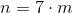. However, this is not sufficient to narrow it down completely.

For example:

If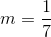, then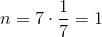, a natural number, putting it in Region I.

If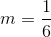, then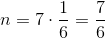, a rational number but not an integer, putting it in Region IV.

From Statement 2 alone, it can be inferred thatis rational, and that it is not an integer. From, it follows that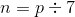. The nonzero rational numbers are closed under division, somust be a rational number. However, sinceis not an integer,cannot be an integer, since the integers are closed under multiplication. Therefore, Statement 2 alone proves thatbelongs in Region IV.

### Example Question #12 : SetsExamine the above diagram, which shows a Venn diagram representing the sets of real numbers.

If real numberwere to be placed in its correct region in the diagram, which one would it be - I, II, III, IV, or V?

Statement 1: If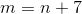, thenwould be placed in Region I.

Statement 2: If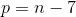, thenwould be placed in Region I.

BOTH statements TOGETHER are sufficient to answer the question, but NEITHER statement ALONE is sufficient to answer the question.

BOTH statements TOGETHER are insufficient to answer the question.

Statement 2 ALONE is sufficient to answer the question, but Statement 1 ALONE is NOT sufficient to answer the question.

EITHER statement ALONE is sufficient to answer the question.

Statement 1 ALONE is sufficient to answer the question, but Statement 2 ALONE is NOT sufficient to answer the question.

Statement 2 ALONE is sufficient to answer the question, but Statement 1 ALONE is NOT sufficient to answer the question.

Explanation:

Region I comprises the natural numbers -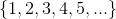From Statement 1 alone,is a natural number; since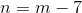, it follows thatis the difference of a natural number and 7 - that is,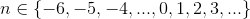could be in any of three regions - I, II, or III.

Conversely, from Statement 2 alone,is the sum of a natural number and 7 - that is,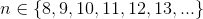must be a natural number and it must be in Region I.

### Example Question #13 : SetsExamine the above diagram, which shows a Venn diagram representing the sets of real numbers.

If real numberwere to be placed in its correct region in the diagram, which one would it be - I, II, III, IV, or V?

Statement 1: If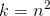, thenwould be in Region I.

Statement 2: If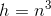, thenwould be in Region III.

BOTH statements TOGETHER are insufficient to answer the question.

BOTH statements TOGETHER are sufficient to answer the question, but NEITHER statement ALONE is sufficient to answer the question.

EITHER statement ALONE is sufficient to answer the question.

Statement 2 ALONE is sufficient to answer the question, but Statement 1 ALONE is NOT sufficient to answer the question.

Statement 1 ALONE is sufficient to answer the question, but Statement 2 ALONE is NOT sufficient to answer the question.

BOTH statements TOGETHER are sufficient to answer the question, but NEITHER statement ALONE is sufficient to answer the question.

Explanation:

Assume Statement 1 alone. It cannot be determined what regionis in.

For example, suppose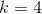, which is in Region I (the set of natural numbers, or positive integers). It is possible that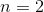, putting it in Region I, or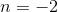, putting it in Region III (the set of integers that are not whole numbers - that is, the set of negative integers).

Assume Statement 2 alone. It cannot be determined what regionis in.

For example, suppose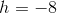, which is in Region III; then, which is also in Region III. But suppose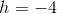; then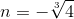, which, as an irrational number, is in Region V.

Now assume both statements. Thenhas an integer as a square and an integer as a cube.must either be an integer or an irrational number. But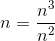, making it the quotient of integers, which is rational. Therefore,is an integer. Furthermore, its cube is negative, sois negative. The two statements together prove thatis a negative integer, which belongs in Region III.

### Example Question #11 : Sets

How many elements are in set?

Statement 1:has exactlysubsets.

Statement 2:has exactlyproper subsets.

Statement 2 ALONE is sufficient to answer the question, but Statement 1 ALONE is NOT sufficient to answer the question.

Statement 1 ALONE is sufficient to answer the question, but Statement 2 ALONE is NOT sufficient to answer the question.

EITHER statement ALONE is sufficient to answer the question.

BOTH statements TOGETHER are insufficient to answer the question.

BOTH statements TOGETHER are sufficient to answer the question, but NEITHER statement ALONE is sufficient to answer the question.

EITHER statement ALONE is sufficient to answer the question.

Explanation:

A set withelements has exactly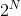subsets in all, and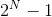proper subsets (every subset except one - the set itself).

From Statement 1, sincehas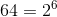subsets, it follows that it has 6 elements. From Statement 2, sincehas 63 proper subsets, it has 64 subsets total, and, again, 6 elements. Either statement alone is sufficient.

### Example Question #15 : Sets

Which, if either, is the greater number:or?

Statement 1: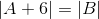Statement 2: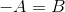EITHER statement ALONE is sufficient to answer the question.

BOTH statements TOGETHER are sufficient to answer the question, but NEITHER statement ALONE is sufficient to answer the question.

BOTH statements TOGETHER are insufficient to answer the question.

Statement 2 ALONE is sufficient to answer the question, but Statement 1 ALONE is NOT sufficient to answer the question.

Statement 1 ALONE is sufficient to answer the question, but Statement 2 ALONE is NOT sufficient to answer the question.

BOTH statements TOGETHER are sufficient to answer the question, but NEITHER statement ALONE is sufficient to answer the question.

Explanation:

Statement 1 alone gives insufficient information. For example, if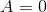, then: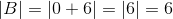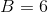or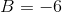Since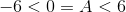, it is unclear which ofandis greater, if either.

Statement 2 gives insufficient information; ifis positive,is negative, and vice versa.

Assume both to be true. The two statements form a system of equations that can be solved using substitution: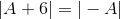Case 1: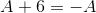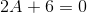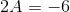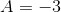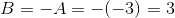Case 2: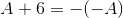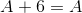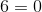This equation has no solution.

Therefore, the only possible solution is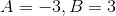. Therefore, it can be concluded that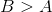.

2 Next →

Tired of practice problems?

Try live online GMAT prep today.